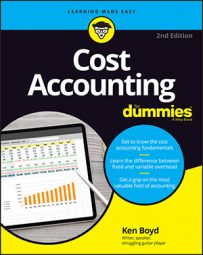##### Cost Accounting For DummiesIn cost accounting, gross margin is defined as sales less cost of sales. Cost of sales isn’t total cost, but the cost to make the good. Gross margin is the price of the asset less the cost to make it. There are other costs (marketing and sales, for example) that aren’t part of the gross margin calculation.

You can express gross margin as a percentage:

Gross margin percentage = gross margin dollar amount ÷ sales x 100

For example, if your gross margin for a pair of shoes is \$10 and your sales price is \$80, your gross margin percentage is 12.5 percent (\$10 ÷ \$80 x 100).

Assume you manufacture leaf blowers. Your two products are heavy-duty blowers and yardwork blowers. You start by computing the gross margin percentage for both of your products together. Total gross margin is \$428,000 (\$3,440,000 in sales value less total costs of \$3,012,000). Here’s the gross margin percentage:

Gross margin percentage = gross margin ÷ total sales value x 100
Gross margin percentage = \$428,000 ÷ \$3,440,000 x 100
Gross margin percentage = 12.442

The gross margin percentage is 12.442 percent.

Now that you have the gross margin percentage for the whole company, you can calculate the gross margin percentage for each product. That amount allows you to come up with the cost of goods available for sale. The table has the details.

Goods Available For Sale by Product
Heavy-Duty Yardwork Total
Sales value (A) \$2,000,000 \$1,440,000 \$3,440,000
Gross margin (B) \$248,837 \$179,163 \$428,000
Cost of goods available for sale (AB) \$1,751,163 \$1,260,837 \$3,012,000

The gross margin for each product equals the sales value multiplied by the gross margin percentage of 12.442 percent. For example, the heavy-duty blower’s gross margin percentage is \$248,837 (2,000,000 x .12442 ÷ 100 with a small rounding difference). Heavy-duty then subtracts the \$248,837 gross margin from \$2,000,000 sales value to get \$1,751,163, the cost of goods available for sale.

Your cost of sales is sales value less gross margin. The formula in the table rearranges the components. Again, you use cost of goods available for sale — at this point, you’re only planning results.